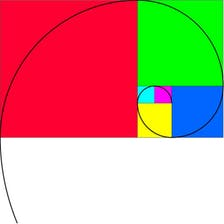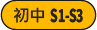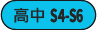# Fibonacci Numbers and the Golden Ratio

HKUSTLearn the mathematics behind the Fibonacci numbers, the golden ratio, and how they are related. These topics are not usually taught in a typical math curriculum, yet contain many fascinating results that are still accessible to an advanced high school student.

The course culminates in an explanation of why the Fibonacci numbers appear unexpectedly in nature, such as the number of spirals in the head of a sunflower.

## Modules

#### Fibonacci: It's as easy as 1, 1, 2, 3

We learn about the Fibonacci numbers, the golden ratio, and their relationship. We derive the celebrated Binet's formula, an explicit formula for the Fibonacci numbers in terms of powers of the golden ratio and its reciprical..

#### Identities, sums and rectangles

We learn about the Fibonacci Q-matrix and Cassini's identity. Cassini's identity is the basis for a famous dissection fallacy colourfully named the Fibonacci bamboozlement. A dissection fallacy is an apparent paradox arising from two arrangements of different area from one set of puzzle pieces. We also derive formulas for the sum of the first n Fibonacci numbers, and the sum of the first n Fibonacci numbers squared. Finally, we show how to construct a golden rectangle, and how this leads to the beautiful image of spiralling squares.

#### The most irrational number

We learn about the golden spiral and the Fibonacci spiral. Because of the relationship between the Fibonacci numbers and the golden ratio, the Fibonacci spiral eventually converges to the golden spiral. You will recognise the Fibonacci spiral because it is the icon of our course. We next learn about continued fractions. To construct a continued fraction is to construct a sequence of rational numbers that converges to a target irrational number. The golden ratio is the irrational number whose continued fraction converges the slowest. We say that the golden ratio is the irrational number that is the most difficult to approximate by a rational number, or that the golden ratio is the most irrational of the irrational numbers. We then define the golden angle, related to the golden ratio, and use it to model the growth of a sunflower head. Use of the golden angle in the model allows a fine packing of the florets, and results in the unexpected appearance of the Fibonacci numbers in the sunflower.

## Course Staff### Jeffrey R. Chasnov

Jeffrey R. Chasnov is a Professor of Mathematics at the Hong Kong University of Science and Technology, where he has been teaching since 1993. He is an expatriate American from New York and California, and earned his BA from UC Berkeley and PhD from Columbia University, with post-doctoral fellowships at NASA, Stanford, and Grenoble, France, and a sabbatical semester at Harvey Mudd College. He is the author of numerous research articles in fluid turbulence and mathematical biology, and has authored online textbooks and videos for his courses on differential equations, matrix algebra, mathematical biology and scientific computation. Before and after work, he enjoys family life, swimming and tennis, and takes great pleasure in his family’s annual camping and skiing vacations.

1. Course Number

fibonacci
2. Classes Start

3. Classes End

Enroll